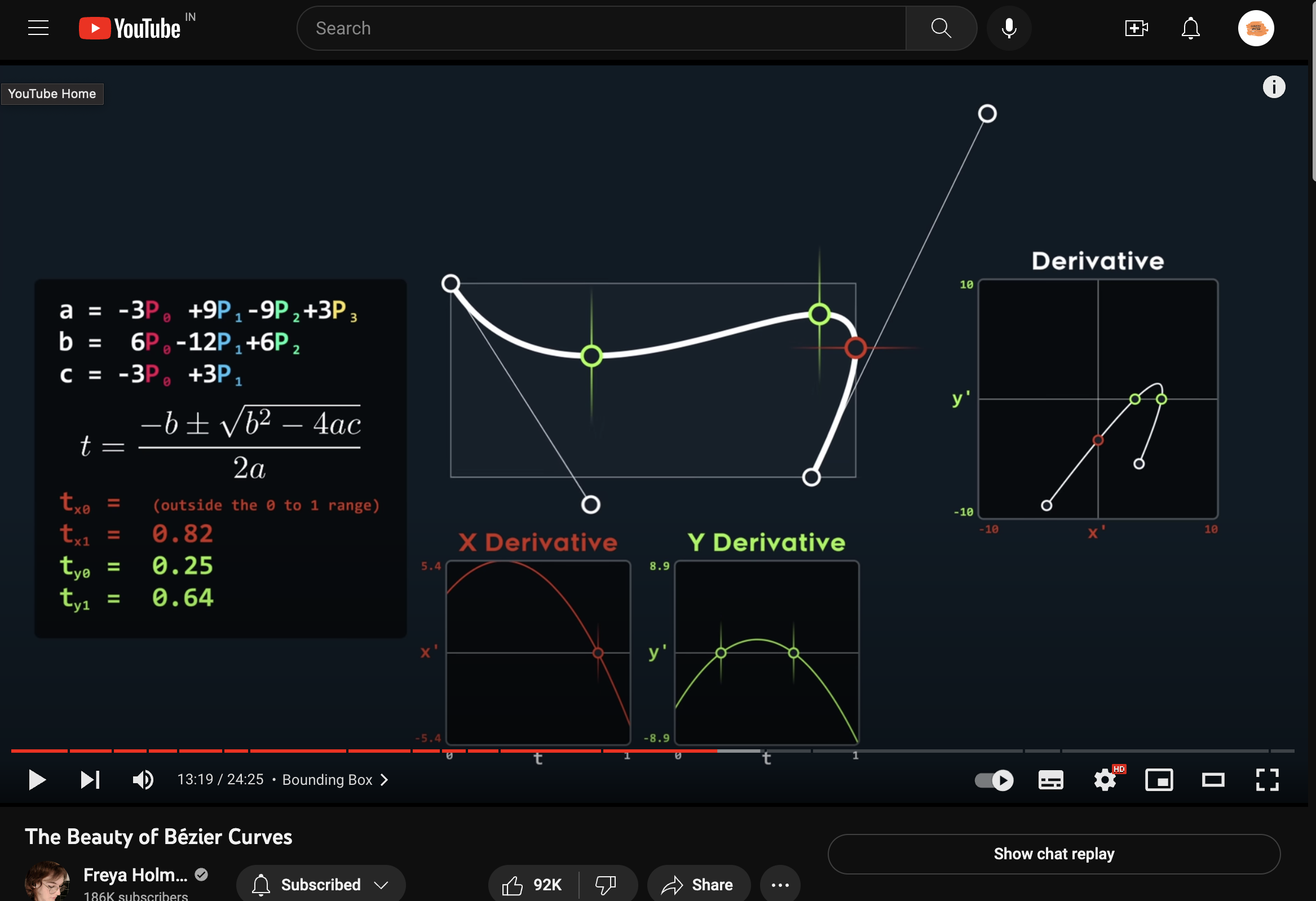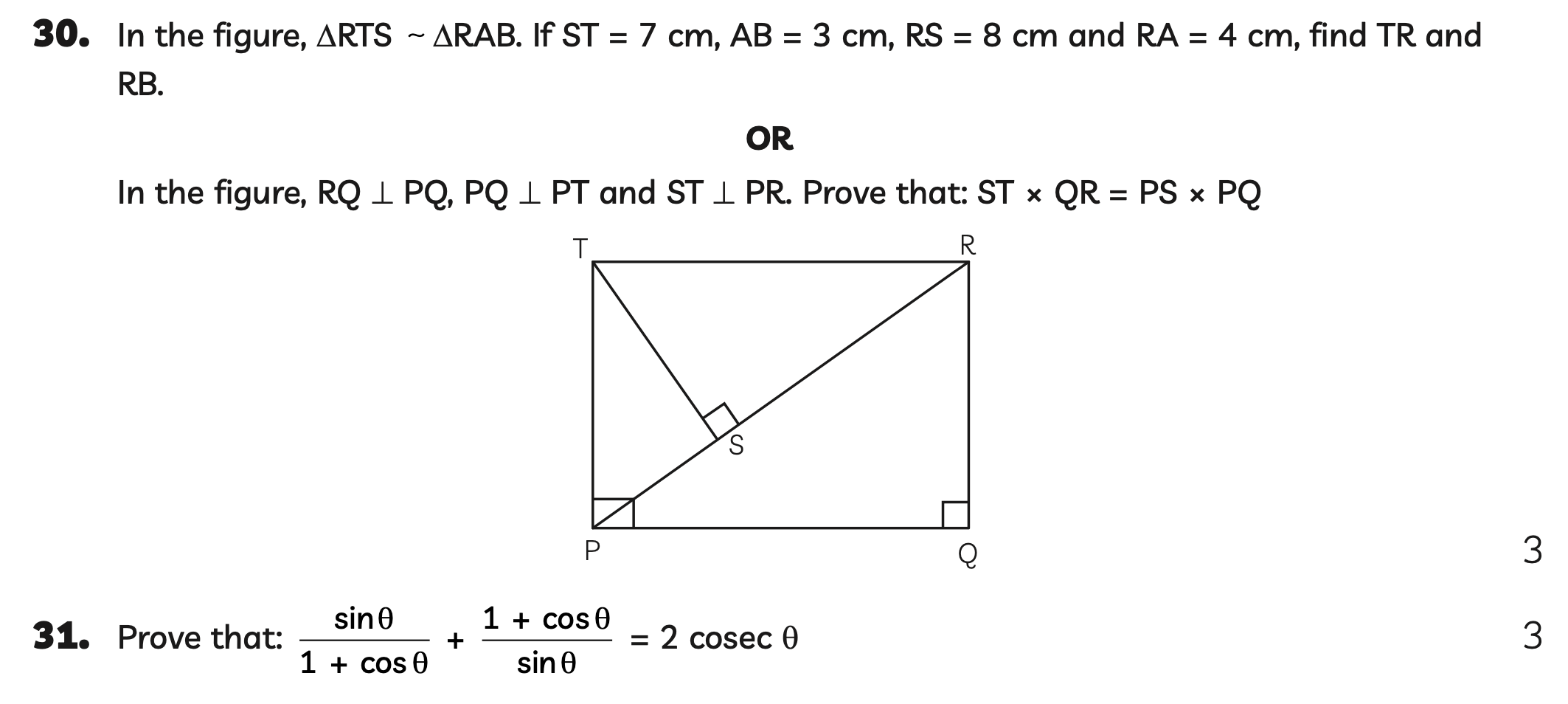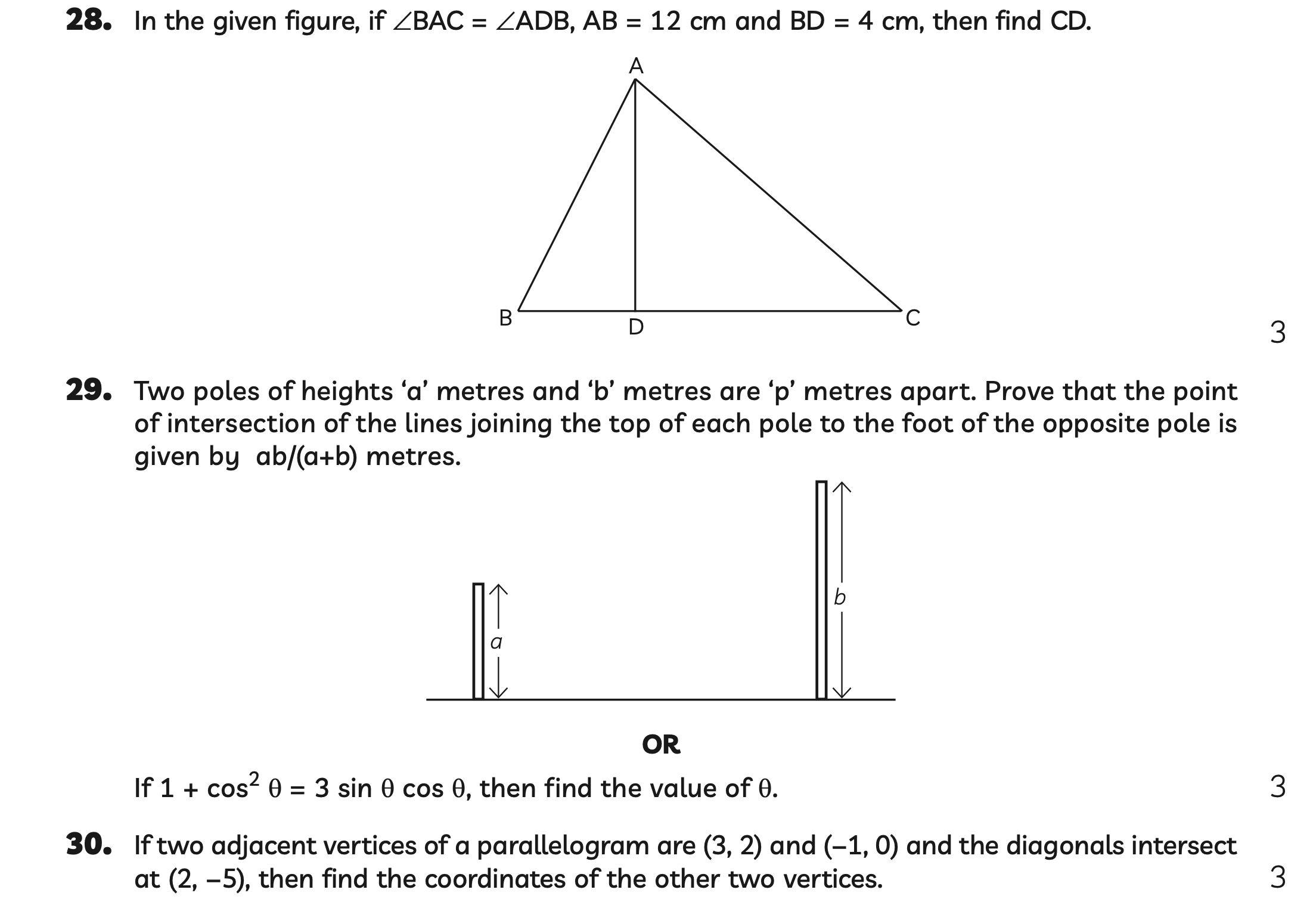Posts tagged with `:math-is-math:`

Watching this masterpiece todaymore math!MATHWhen you transform `f(x)=e^x` 1 unit to the left, you’re left with `g(x)=e^(x+1)` (Fig. A). This transformation is pretty simple; the new line is 1 unit away. However, it’s only 1 unit away horizontally. Vertically, it varies depending on the x value. The vertical difference at x=-1 is a lot smaller than the vertical difference at x=1. On this recent #hack-night, @carrot and I set out to transform `f(x)` so that at any point along `f(x)`, the distance to the closest point on `g(x)` is equal to 1. Essentially, `g(x)` is 1 unit away throughout, not horizontally (Fig. B). This proved to be quite difficult. There were a lot of quadratics that needed solving, and we also had to use the pythagorean theorem to get some distances. He can probably explain the math behind it a lot better than I can. Anyways, here are a few links if you want to try it out: • Exponential Functions - www.desmos.com/calculator/euke7u53si • Quadratic Functions - www.desmos.com/calculator/jekjpm1mj0 • Linear Functions - www.desmos.com/calculator/oljptwxhji • Circles - www.desmos.com/calculator/uedqiqjjxk (I think)
\day 6\ math is gonna kill me someday. died on math hw today. don’t have anything to attach so here’s a picture showing my disdain. :math-is-math: 🔪Day 2 of #10-days-in-public, walked through some derivations of important equations in QM, and fixed my simulation a bitadded a complex step-by-step math API to my website, which basically just screenshots and restyles cymath to get a visual answer~Gotta new notebook for homeworksl~:absolutelynot:Did a lot of Club work, here is a question from the recruitment form, think you can answer?